# Uncoupled Regression from Pairwise Comparison Data

Sep. 17, 2020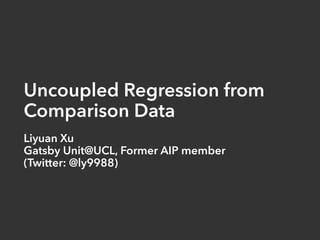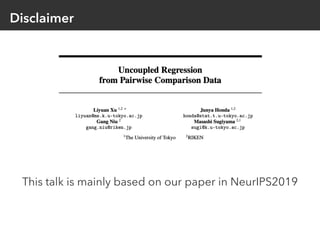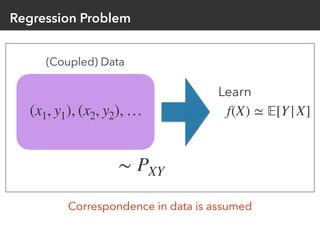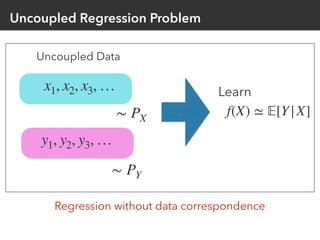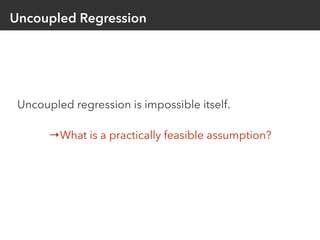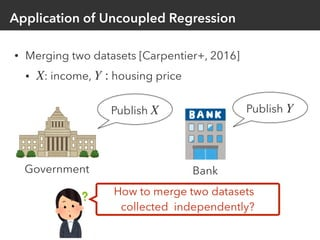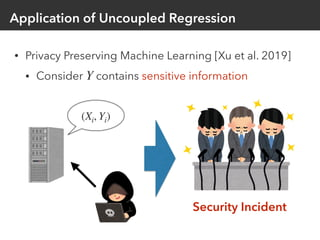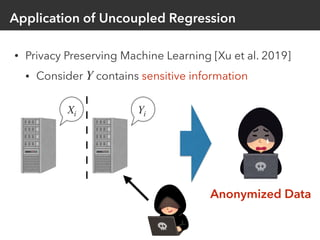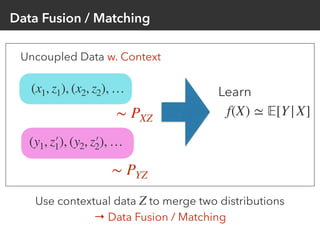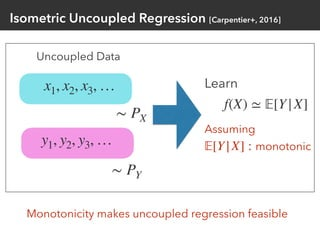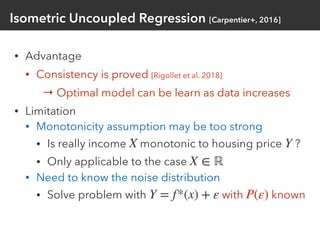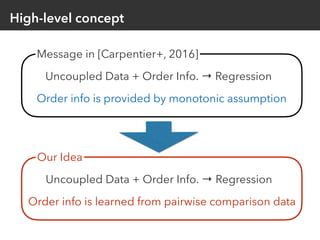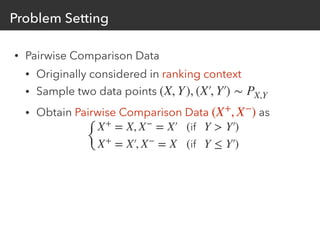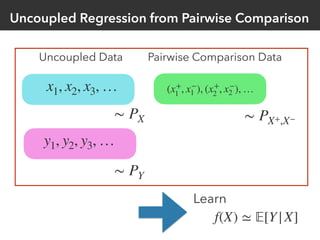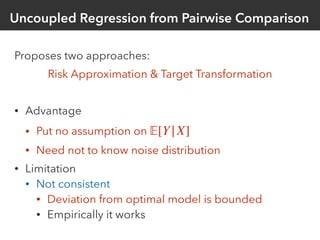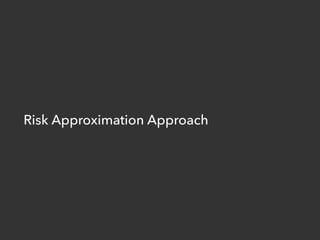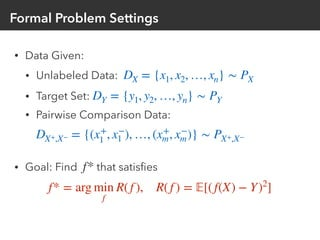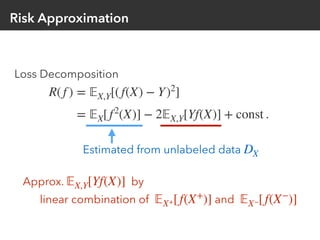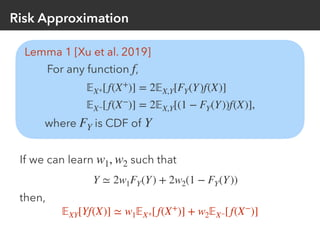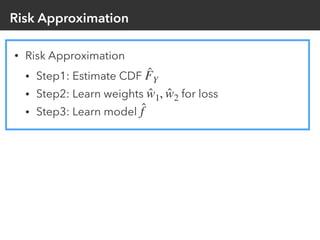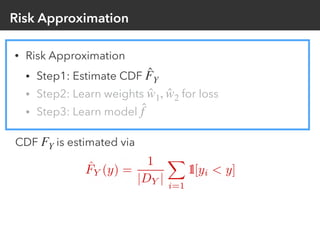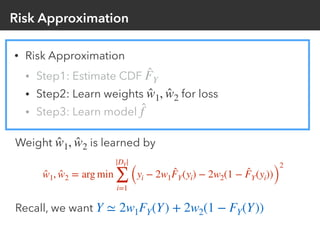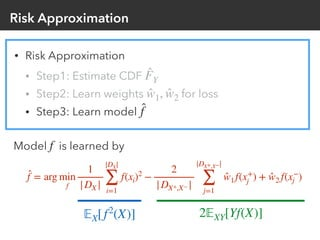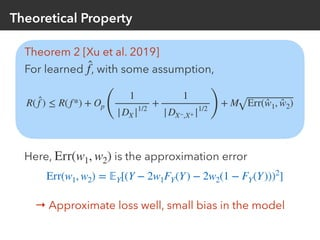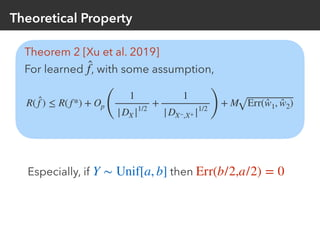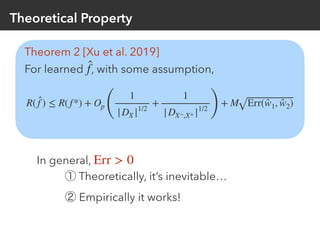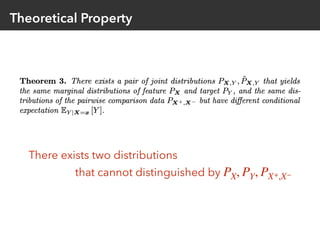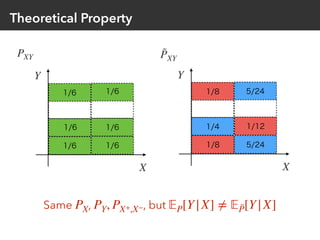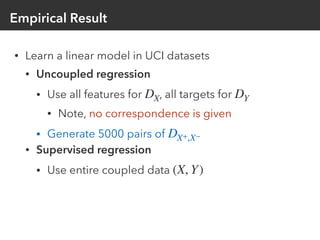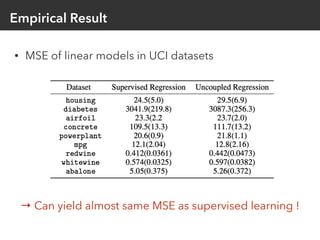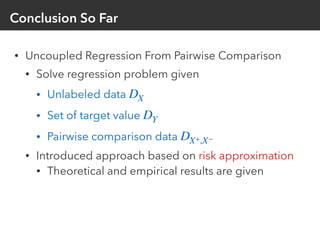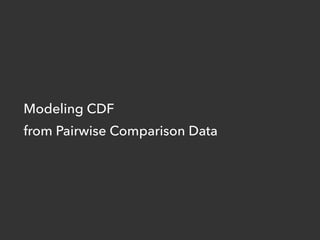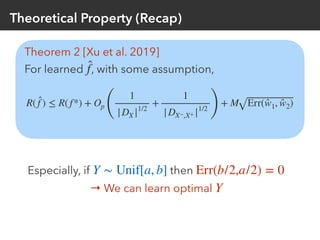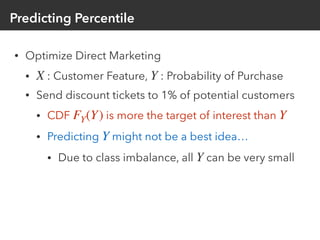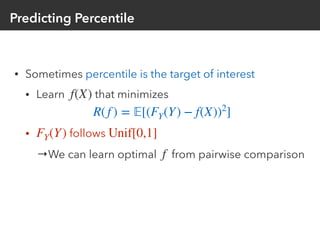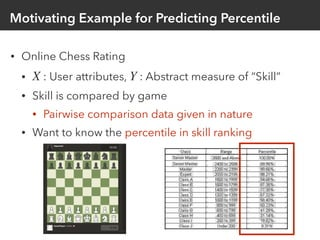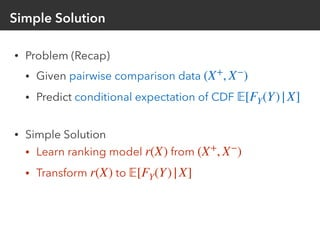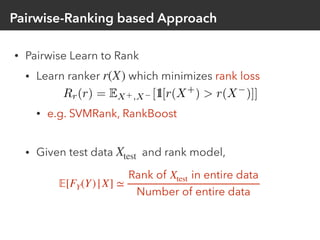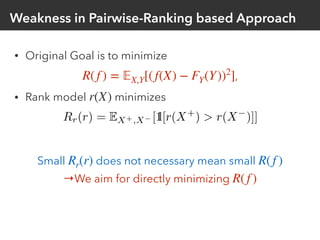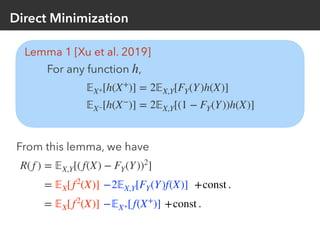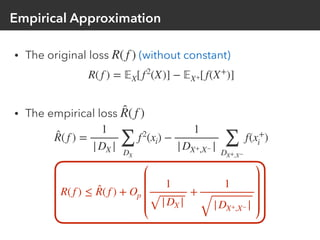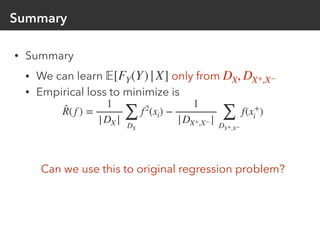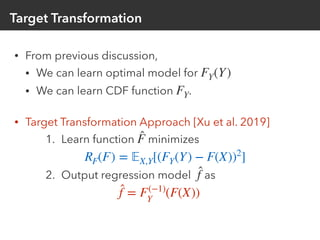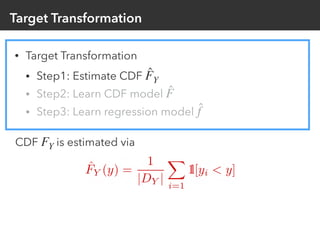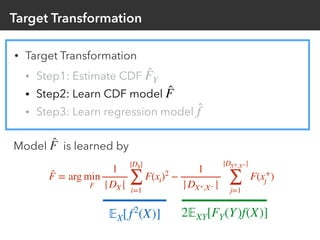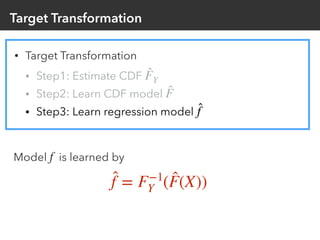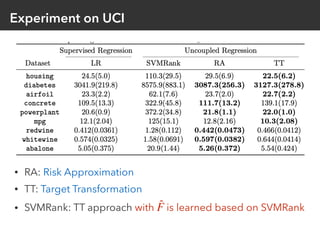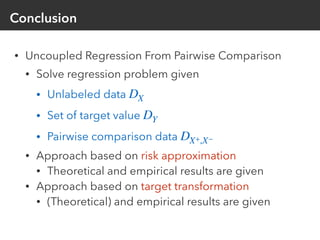1 of 52

### Uncoupled Regression from Pairwise Comparison Data

• 1. Uncoupled Regression from Comparison Data Liyuan Xu Gatsby Unit@UCL, Former AIP member (Twitter: @ly9988)
• 2. Disclaimer This talk is mainly based on our paper in NeurIPS2019
• 3. Introduction
• 4. Regression Problem (x1, y1), (x2, y2), … (Coupled) Data ∼ PXY f(X) ≃ 𝔼[Y|X] Learn Correspondence in data is assumed
• 5. Uncoupled Regression Problem Uncoupled Data ∼ PX x1, x2, x3, … ∼ PY y1, y2, y3, … f(X) ≃ 𝔼[Y|X] Learn Regression without data correspondence
• 6. Uncoupled Regression Uncoupled regression is impossible itself. →What is a practically feasible assumption?
• 7. Application of Uncoupled Regression • Merging two datasets [Carpentier+, 2016] • : income, housing priceX Y : Government Publish X Bank Publish Y How to merge two datasets collected independently?
• 8. Application of Uncoupled Regression • Privacy Preserving Machine Learning [Xu et al. 2019] • Consider contains sensitive informationY (Xi, Yi) Security Incident
• 9. Application of Uncoupled Regression • Privacy Preserving Machine Learning [Xu et al. 2019] • Consider contains sensitive informationY Xi Yi Anonymized Data
• 10. Data Fusion / Matching Uncoupled Data w. Context ∼ PXZ (x1, z1), (x2, z2), … ∼ PYZ (y1, z′1), (y2, z′2), … f(X) ≃ 𝔼[Y|X] Learn Use contextual data to merge two distributions → Data Fusion / Matching Z
• 11. Isometric Uncoupled Regression [Carpentier+, 2016] Uncoupled Data ∼ PX x1, x2, x3, … ∼ PY y1, y2, y3, … f(X) ≃ 𝔼[Y|X] Learn Assuming 𝔼[Y|X] : monotonic Monotonicity makes uncoupled regression feasible
• 12. Isometric Uncoupled Regression [Carpentier+, 2016] • Advantage • Consistency is proved [Rigollet et al. 2018] → Optimal model can be learn as data increases • Limitation • Monotonicity assumption may be too strong • Is really income monotonic to housing price ? • Only applicable to the case • Need to know the noise distribution • Solve problem with with known X Y X ∈ ℝ Y = f*(x) + ε P(ε)
• 13. High-level concept Message in [Carpentier+, 2016] Uncoupled Data + Order Info. → Regression Order info is provided by monotonic assumption Our Idea Order info is learned from pairwise comparison data Uncoupled Data + Order Info. → Regression
• 14. Problem Setting • Pairwise Comparison Data • Originally considered in ranking context • Sample two data points • Obtain Pairwise Comparison Data as (X, Y), (X′, Y′) ∼ PX,Y (X+ , X− ) { X+ = X, X− = X′ (if Y > Y′) X+ = X′, X− = X (if Y ≤ Y′)
• 15. Uncoupled Regression from Pairwise Comparison ∼ PX x1, x2, x3, … ∼ PY y1, y2, y3, … f(X) ≃ 𝔼[Y|X] Learn ∼ PX+,X− (x+ 1 , x− 1 ), (x+ 2 , x− 2 ), … Uncoupled Data Pairwise Comparison Data
• 16. Uncoupled Regression from Pairwise Comparison Proposes two approaches: Risk Approximation & Target Transformation • Advantage • Put no assumption on • Need not to know noise distribution • Limitation • Not consistent • Deviation from optimal model is bounded • Empirically it works 𝔼[Y|X]
• 17. Risk Approximation Approach
• 18. Formal Problem Settings • Data Given: • Unlabeled Data: • Target Set: • Pairwise Comparison Data: • Goal: Find that satisﬁes DX = {x1, x2, …, xn} ∼ PX DY = {y1, y2, …, yn} ∼ PY DX+,X− = {(x+ 1 , x− 1 ), …, (x+ m, x− m)} ∼ PX+,X− f* f* = arg min f R(f ), R(f ) = 𝔼[(f(X) − Y)2 ]
• 19. Risk Approximation Loss Decomposition R(f ) = 𝔼X,Y[(f(X) − Y)2 ] = 𝔼X[f2 (X)] − 2𝔼X,Y[Yf(X)] + const . Estimated from unlabeled data DX Approx. by linear combination of and 𝔼X,Y[Yf(X)] 𝔼X+[f(X+ )] 𝔼X−[f(X− )]
• 20. Risk Approximation Lemma 1 [Xu et al. 2019] For any function ,f 𝔼X+[f(X+ )] = 2𝔼X,Y[FY(Y)f(X)] 𝔼X−[f(X− )] = 2𝔼X,Y[(1 − FY(Y))f(X)], where is CDF ofFY Y If we can learn such thatw1, w2 Y ≃ 2w1FY(Y) + 2w2(1 − FY(Y)) then, 𝔼XY[Yf(X)] ≃ w1 𝔼X+[f(X+ )] + w2 𝔼X−[f(X− )]
• 21. Risk Approximation • Risk Approximation • Step1: Estimate CDF • Step2: Learn weights for loss • Step3: Learn model ̂FY ̂w1, ̂w2 ̂f
• 22. Risk Approximation • Risk Approximation • Step1: Estimate CDF • Step2: Learn weights for loss • Step3: Learn model ̂FY ̂w1, ̂w2 ̂f CDF is estimated viaFY
• 23. Risk Approximation • Risk Approximation • Step1: Estimate CDF • Step2: Learn weights for loss • Step3: Learn model ̂FY ̂w1, ̂w2 ̂f Weight is learned bŷw1, ̂w2 ̂w1, ̂w2 = arg min |DY| ∑ i=1 (yi − 2w1 ̂FY(yi) − 2w2(1 − ̂FY(yi))) 2 Recall, we want Y ≃ 2w1FY(Y) + 2w2(1 − FY(Y))
• 24. Risk Approximation • Risk Approximation • Step1: Estimate CDF • Step2: Learn weights for loss • Step3: Learn model ̂FY ̂w1, ̂w2 ̂f Model is learned byf ̂f = arg min f 1 |DX | |DX| ∑ i=1 f(xi)2 − 2 |DX+,X− | |DX+,X−| ∑ j=1 ̂w1f(x+ j ) + ̂w2 f(x− j ) 𝔼X[f2 (X)] 2𝔼XY[Yf(X)]
• 25. Theoretical Property Theorem 2 [Xu et al. 2019] For learned , with some assumption,̂f R( ̂f ) ≤ R(f*) + Op ( 1 |DX |1/2 + 1 |DX−,X+ |1/2 ) + M Err( ̂w1, ̂w2) Here, is the approximation errorErr(w1, w2) Err(w1, w2) = 𝔼Y[(Y − 2w1FY(Y) − 2w2(1 − FY(Y)))2 ] → Approximate loss well, small bias in the model
• 26. Theoretical Property Theorem 2 [Xu et al. 2019] For learned , with some assumption,̂f Especially, if thenY ∼ Unif[a, b] Err(b/2,a/2) = 0 R( ̂f ) ≤ R(f*) + Op ( 1 |DX |1/2 + 1 |DX−,X+ |1/2 ) + M Err( ̂w1, ̂w2)
• 27. Theoretical Property Theorem 2 [Xu et al. 2019] For learned , with some assumption,̂f In general, ① Theoretically, it’s inevitable… ② Empirically it works! Err > 0 R( ̂f ) ≤ R(f*) + Op ( 1 |DX |1/2 + 1 |DX−,X+ |1/2 ) + M Err( ̂w1, ̂w2)
• 28. Theoretical Property There exists two distributions that cannot distinguished by PX, PY, PX+,X−
• 29. Theoretical Property PXY X Y ˜PXY X Y 1/6 1/8 5/24 1/4 1/8 5/24 1/6 1/6 1/6 1/6 1/6 1/12 Same , , butPX PY, PX+,X− 𝔼P[Y|X] ≠ 𝔼 ˜P[Y|X]
• 30. Empirical Result • Learn a linear model in UCI datasets • Uncoupled regression • Use all features for , all targets for • Note, no correspondence is given • Generate 5000 pairs of • Supervised regression • Use entire coupled data DX DY DX+,X− (X, Y)
• 31. Empirical Result • MSE of linear models in UCI datasets → Can yield almost same MSE as supervised learning !
• 32. Conclusion So Far • Uncoupled Regression From Pairwise Comparison • Solve regression problem given • Unlabeled data • Set of target value • Pairwise comparison data • Introduced approach based on risk approximation • Theoretical and empirical results are given DX DY DX+,X−
• 33. Modeling CDF from Pairwise Comparison Data
• 34. Theoretical Property (Recap) Theorem 2 [Xu et al. 2019] For learned , with some assumption,̂f Especially, if then → We can learn optimal Y ∼ Unif[a, b] Err(b/2,a/2) = 0 Y R( ̂f ) ≤ R(f*) + Op ( 1 |DX |1/2 + 1 |DX−,X+ |1/2 ) + M Err( ̂w1, ̂w2)
• 35. Predicting Percentile • Optimize Direct Marketing • : Customer Feature, : Probability of Purchase • Send discount tickets to 1% of potential customers • CDF is more the target of interest than • Predicting might not be a best idea… • Due to class imbalance, all can be very small X Y FY(Y) Y Y Y
• 36. Predicting Percentile • Sometimes percentile is the target of interest • Learn that minimizes • follows →We can learn optimal from pairwise comparison f(X) R(f ) = 𝔼[(FY(Y) − f(X))2 ] FY(Y) Unif[0,1] f
• 37. Motivating Example for Predicting Percentile • Online Chess Rating • : User attributes, : Abstract measure of “Skill” • Skill is compared by game • Pairwise comparison data given in nature • Want to know the percentile in skill ranking X Y
• 38. Simple Solution • Problem (Recap) • Given pairwise comparison data • Predict conditional expectation of CDF • Simple Solution • Learn ranking model from • Transform to (X+ , X− ) 𝔼[FY(Y)|X] r(X) (X+ , X− ) r(X) 𝔼[FY(Y)|X]
• 39. Pairwise-Ranking based Approach • Pairwise Learn to Rank • Learn ranker which minimizes rank loss • e.g. SVMRank, RankBoost • Given test data and rank model, r(X) Xtest 𝔼[FY(Y)|X] ≃ Rank of Xtest in entire data Number of entire data
• 40. Weakness in Pairwise-Ranking based Approach • Original Goal is to minimize , • Rank model minimizes Small does not necessary mean small →We aim for directly minimizing R(f ) = 𝔼X,Y[(f(X) − FY(Y))2 ] r(X) Rr(r) R(f ) R(f )
• 41. Direct Minimization Lemma 1 [Xu et al. 2019] For any function ,h 𝔼X+[h(X+ )] = 2𝔼X,Y[FY(Y)h(X)] 𝔼X−[h(X− )] = 2𝔼X,Y[(1 − FY(Y))h(X)] From this lemma, we have R(f ) = 𝔼X,Y[(f(X) − FY(Y))2 ] = 𝔼X[f2 (X)] −2𝔼X,Y[FY(Y)f(X)] +const . = 𝔼X[f2 (X)] −𝔼X+[f(X+ )] +const .
• 42. R(f ) ≤ ̂R(f ) + Op 1 |DX | + 1 |DX+,X− | Empirical Approximation • The original loss (without constant) • The empirical loss R(f ) R(f ) = 𝔼X[f2 (X)] − 𝔼X+[f(X+ )] ̂R(f ) ̂R(f ) = 1 |DX | ∑ DX f2 (xi) − 1 |DX+,X− | ∑ DX+,X− f(x+ i )
• 43. Summary • Summary • We can learn only from • Empirical loss to minimize is Can we use this to original regression problem? 𝔼[FY(Y)|X] DX, DX+,X− ̂R(f ) = 1 |DX | ∑ DX f2 (xi) − 1 |DX+,X− | ∑ DX+,X− f(x+ i )
• 44. Target Transform Approach
• 45. Target Transformation • From previous discussion, • We can learn optimal model for • We can learn CDF function . • Target Transformation Approach [Xu et al. 2019] 1. Learn function minimizes 2. Output regression model as FY(Y) FY ̂F RF(F) = 𝔼X,Y[(FY(Y) − F(X))2 ] ̂f ̂f = F(−1) Y (F(X))
• 46. Target Transformation • Target Transformation • Step1: Estimate CDF • Step2: Learn CDF model • Step3: Learn regression model ̂FY ̂F ̂f
• 47. Target Transformation • Target Transformation • Step1: Estimate CDF • Step2: Learn CDF model • Step3: Learn regression model ̂FY ̂F ̂f CDF is estimated viaFY
• 48. Target Transformation • Target Transformation • Step1: Estimate CDF • Step2: Learn CDF model • Step3: Learn regression model ̂FY ̂F ̂f Model is learned bŷF ̂F = arg min F 1 |DX | |DX| ∑ i=1 F(xi)2 − 1 |DX+,X− | |DX+,X−| ∑ j=1 F(x+ j ) 𝔼X[f2 (X)] 2𝔼XY[FY(Y)f(X)]
• 49. Target Transformation • Target Transformation • Step1: Estimate CDF • Step2: Learn CDF model • Step3: Learn regression model ̂FY ̂F ̂f Model is learned byf ̂f = F−1 Y ( ̂F(X))
• 50. Experiment on UCI • RA: Risk Approximation • TT: Target Transformation • SVMRank: TT approach with is learned based on SVMRank̂F
• 51. Conclusion • Uncoupled Regression From Pairwise Comparison • Solve regression problem given • Unlabeled data • Set of target value • Pairwise comparison data • Approach based on risk approximation • Theoretical and empirical results are given • Approach based on target transformation • (Theoretical) and empirical results are given DX DY DX+,X−